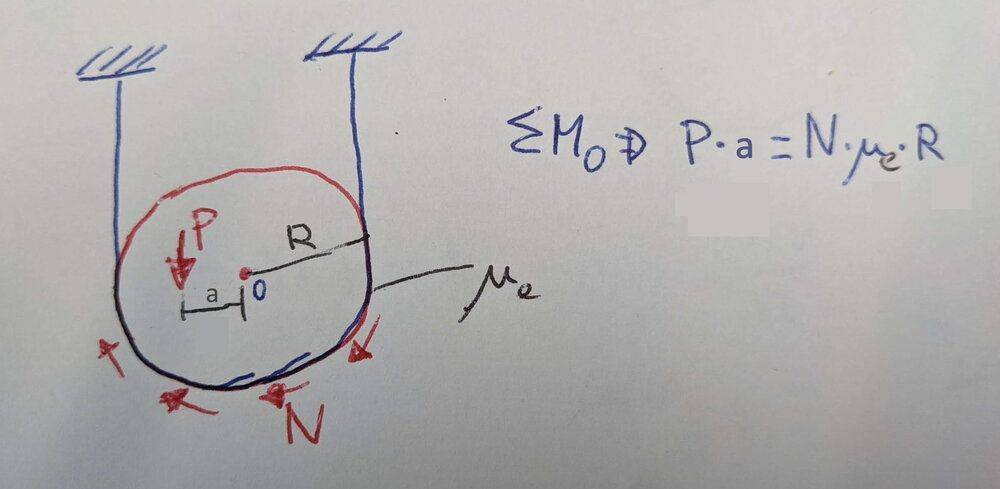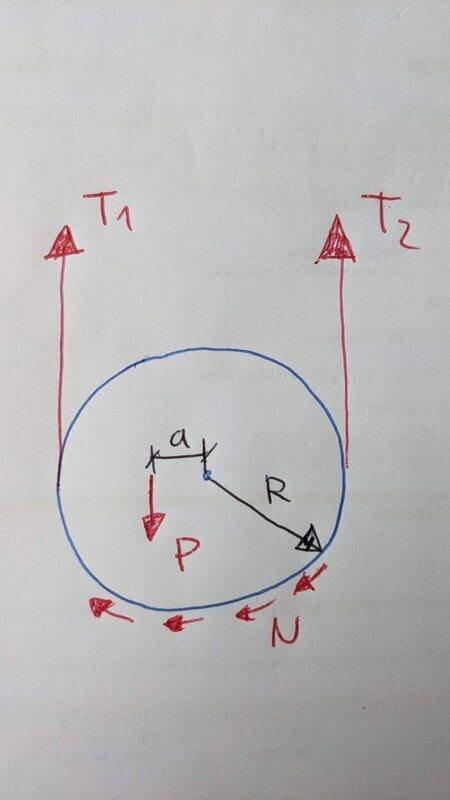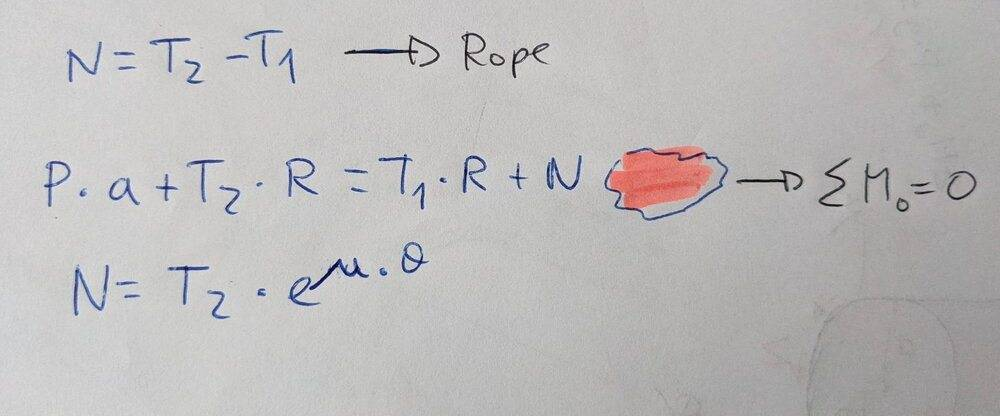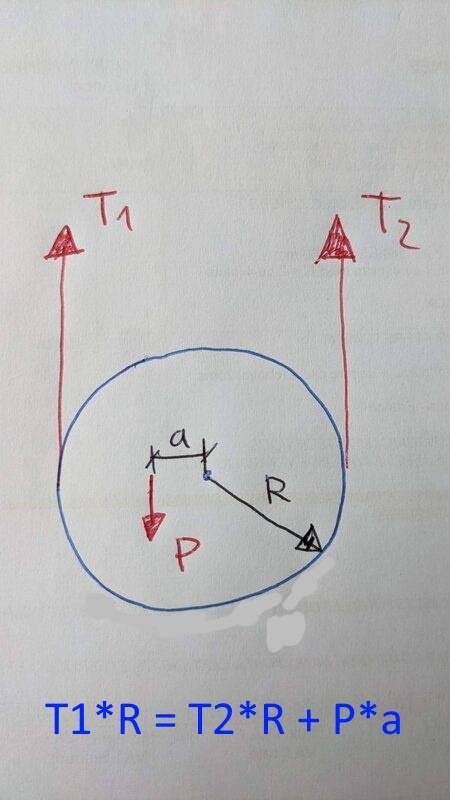# Friction of a rope around a cylinder

davidgruty
Hi,

We have a cylinder held by a rope . The rope holds it around its lower half.

If the center of mass of the cylinder is not in the middle, but towards a side, a distance "a". How does this offset affect to the friction on the rope?

or

How big does "a" have to be so the cylinder starts moving?

The weight of the cylinder "P" goes downwards. But the friction is along the lower half of it. Can one sum the moments around the center?

P*a=N*mue*R {1}

N: reaction force along the rope
mue: static friction coeficient

In general:

P=mue*N {2}

I can't combine these two

How can I calculate "a"?thanks

Gold Member
I can't derive the formula, but remember from a school boy that the frictional resistance obtained by wrapping a rope around a cylinder is as follows:-
Frictional resistance = Highest Tension x e^(mu x theta).
I notice your formula does not include the tension, which will vary along the wrapping.

davidgruty
ok, right. I just realized the the tension in both sides of the rope is not the same

davidgruty
What is theta? the angle of contact between rope and cylinder? 180º in this case (Pi)

So

N = T2 * e^(mu*PI)

?

There are 4 forces: the two tensions (T1, T2), the friction (N) and the weight (P)

How can I connect them? I can't say that the sum of forces in the vertical axis is zero. N is changing directionHomework Helper
If the center of mass of the cylinder is not in the middle, but towards a side, a distance "a". How does this offset affect to the friction on the rope?
The first question to be answered is whether the rope is slipping.

The standard way of doing this is to start by assuming that the rope is not slipping and to then determine the forces that must exist everywhere and the resulting accelerations. If the coefficient of static friction is inadequate to provide the required force of static friction then one concludes that the rope is slipping.

If the rope is slipping, the coefficient of kinetic friction can be used to deduce the pattern of frictional force that must exist and one can then deduce the acceleration that exists.

davidgruty
Yes, the rope should not slip.

I want to find the distance "a" maximum before starts slipping.

Using the static friction coefficient

Homework Helper
Use the formula provided by @tech99 in post #2. If the rope is on the verge of slipping, that formula gives the pattern of tension that must exist. In particular, for a given coefficient of friction, it can be solved for T1 in terms of T2. Or for T2 in terms of T1.

The rest is a matter of writing down equations and solving.

davidgruty
I'm not following. What do you mean with T1 in terms of T2? their relation?

Such
T2 = N + T1
?

This is one equation on the rope

Then we have the

N = T2 * e^(mu*theta)

And finally the moments around the center:

P * a + T2 * R = T1 * R + N (xxxx)

I don't know how N contributes in the formula. I am guessing N * R * theta (the force along its contact line times the distance to the center)

I can't find in internet the formula Frictional resistance = Highest Tension x e^(mu x theta)

Could you address me to some theory or examples where it is applied?

thanksHomework Helper
Gold Member
https://en.m.wikipedia.org/wiki/Capstan_equation

Note that as distance a gets bigger, two things also grow: the value of the moment excerted by off-center force P and the difference between tensions of the the rope.
In real life, force P would move the cylinder in the diagram towards the right some, as a state of static balance appears.

Homework Helper
https://en.m.wikipedia.org/wiki/Capstan_equation

Note that as distance a gets bigger, two things also grow: the value of the moment excerted by off-center force P and the difference between tensions of the the rope.
In real life, force P would move the cylinder in the diagram towards the right some, as a state of static balance appears.
I agree with the use of the capstan equation. I disagree that there will be any rightward slant. Consider that in a state of static equilibrium if both tensions had a rightward component and the downward force of gravity were still purely vertical, this would give a net rightward force, contradicting the claim that we have a static equilibrium.

I hesitate to give a full solution to this homework-like problem. It is dead easy.

davidgruty
ahá,

I see now well the equation. So I have the relation between T1 and T2.

Now I need to plug it in the equilibrium equations, but I don't see them yet.

How does the friction force affect the cylinder??

Homework Helper
ahá,

I see now well the equation. So I have the relation between T1 and T2.

Now I need to plug it in the equilibrium equations, but I don't see them yet.

How does the friction force affect the cylinder??
It is most convenient to pretend that the section of cord between the two attachment points is part and parcel of the cylinder. That segment will be negligibly massive and will move with the cylinder, so this interpretation does not change the problem in any meaningful way.

[You use the capstan equation to determine the ratio of the tensions first, then apply this re-interpretation].

With this new interpretation in hand, the cylinder is subject to three external forces and friction is no longer relevant.

davidgruty
ok!

So sum of moments respect to the center and I already have it.

Like this:davidgruty
or just sum of forces in the vertical direction:

T1 + T2 = P